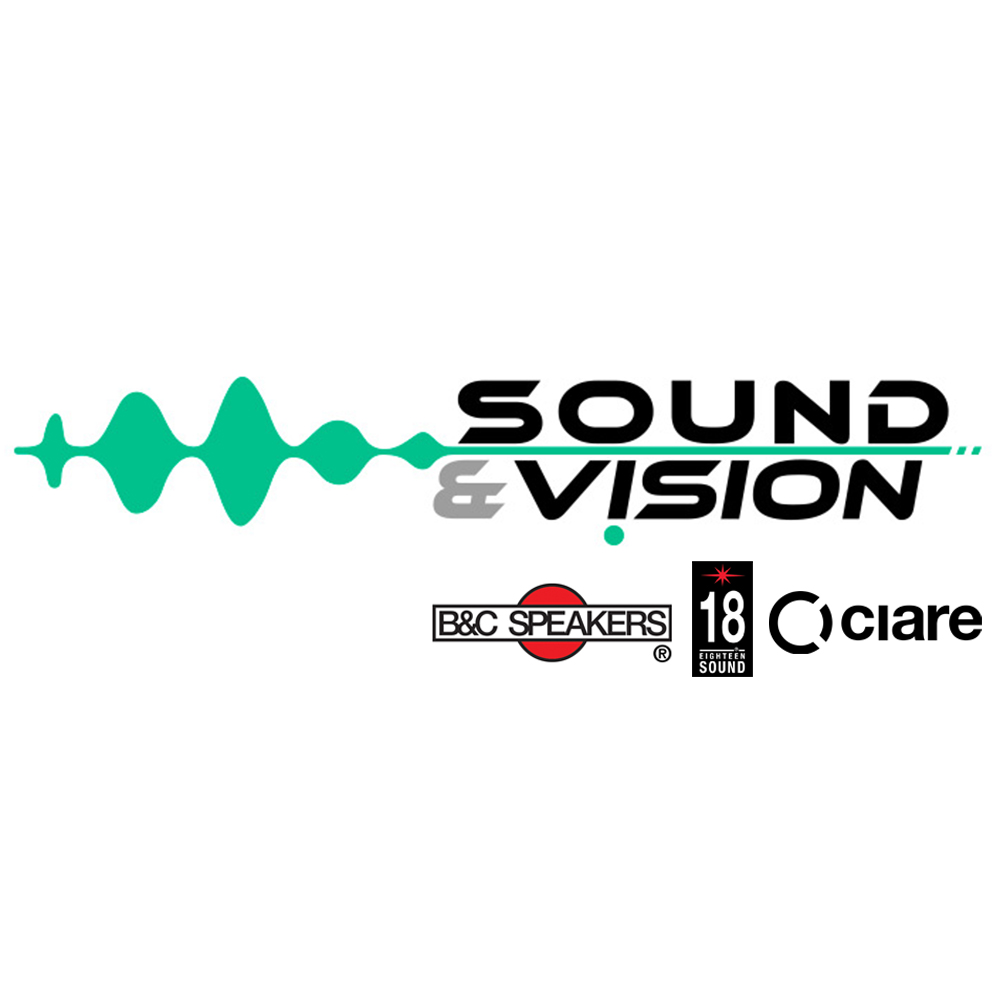## NDI12.50W 8 Ohm 4 Ohm### Woofer 12'' Neodimio

Program Power
Rated Impedance
Nominal Diameter
Sensitivity
Voice Coil Diameter
Frequency Range
450 W
8 Ohm
12''- 320 mm
97,5 dB (2,83V/1m)
2 in - 50 mm
50-4000 Hz

#### Notes

1 Nominal power is determined according to AES2-1984 (r2003) standard.
2 Program Power is defined as 3 dB greater than the Nominal rating.
3 Sensitivity represents the averaged value of acoustic output as measured on the forward central axis of cone, at distance 1m, when connected to 2,83V sine wave test signal.
4 Frequency range is given as the band of frequencies delineated by the lower and upper limits where the output level drops by 10 dB below the rated sensitivity in half space environment.
5 Linear Math. Xmax is calculated as (Hvc-Hg)/2 + Hg/4 where Hvc is the coil depth and Hg is the gapdepth.
6Frequency response curve In the range above 150 Hz is measured on infinite baffle conditions and simulated as per recommended loading in the range below 150 Hz.
7 Impedance curve is measured in free air conditions at small signals.